Finite Mathematics : Linear Inequalities and Linear Programming

Example Questions

Example Question #1 : Linear Inequalities And Linear Programming

The graph depicts which inequality?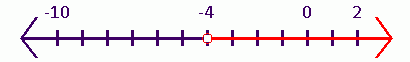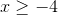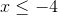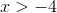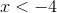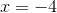Explanation:

To identify the inequality the graph represents first identify range.Since the red line goes from negative four to infinity, we can right this in mathematical terms as follows,Now, the inequality will remain a greater than sign because there is an open circle at four.

This means thatdoes not equal four, it only equals values greater than four.

Thus, the correct inequality isExample Question #31 : Finite Mathematics

The initial simplex tableau for a maximization linear programming problem is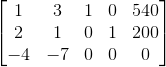Locate the first pivot element.

Row 1, Column 2

Row 2, Column 2

Row 2, Column 1

Row 1, Column 1

Row 1, Column 2

Explanation:

To select the pivot element while working a maximization problem, first, identify the pivot column by selecting the "most negative" element in the bottom, or objective, row. In the initial tableau, it is thein Column 2: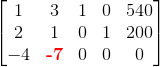In the other rows, divide the rightmost element by the element in the pivot column. The pivot row is identified by selecting the "least positive" quotient.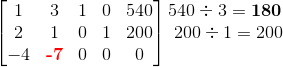The least positive quotient appears in Row 1. This puts the pivot element in Row 1, Column 2.